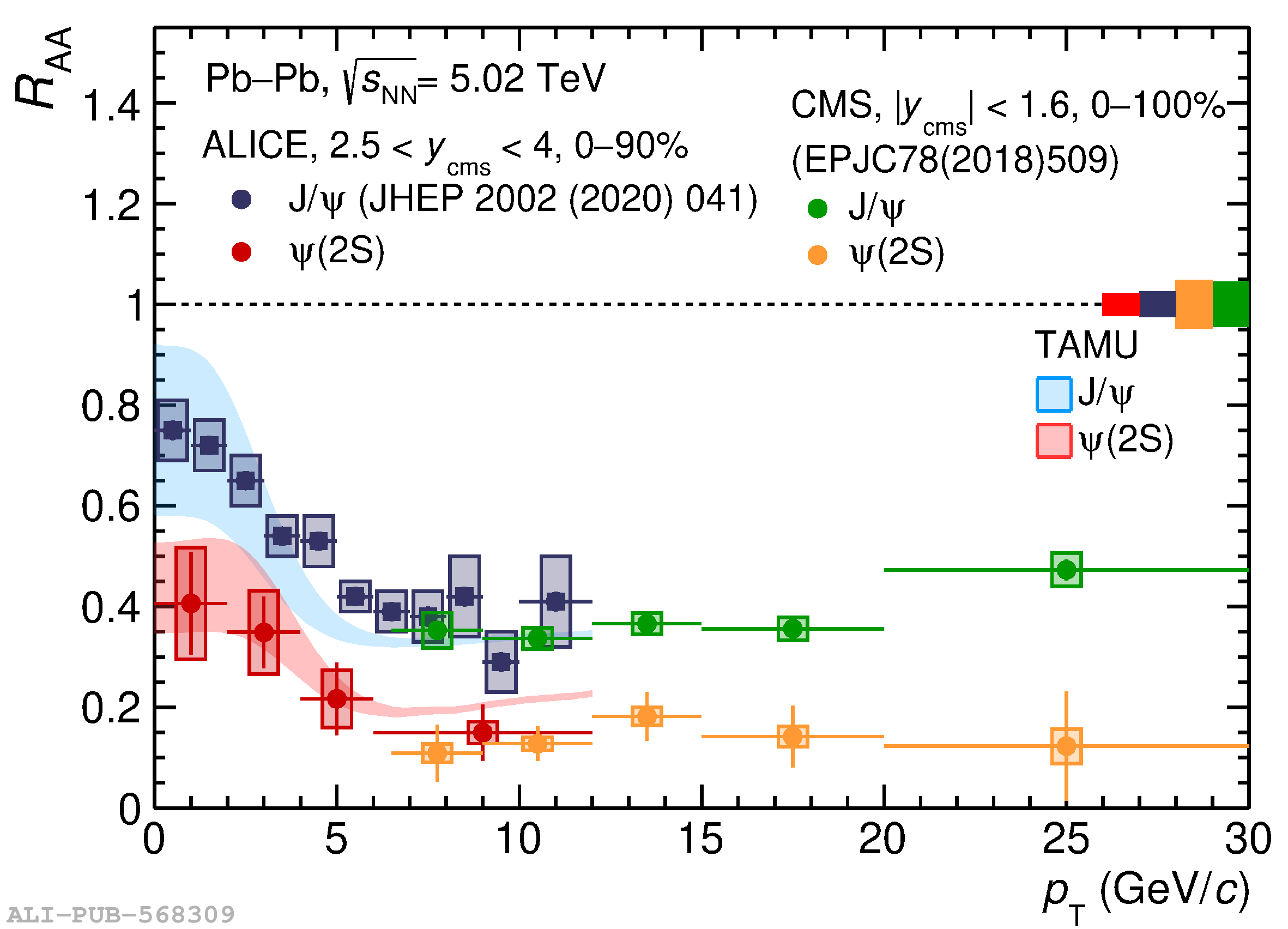# Figure 4

 The $R_{\rm AA}$ for $\psi({\rm 2S})$ and J/$\psi$  as a function of $p_{\rm T}$. Comparison with theory models and results from the CMS experiment  are also shown.